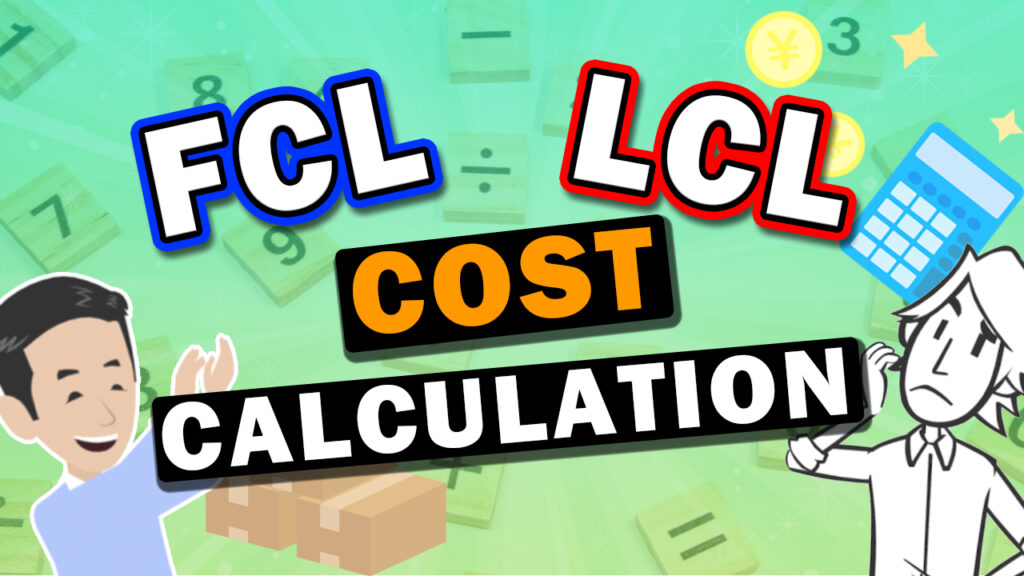Neko Senpai

## Explained about LCL Cargo Transportation by VideoKamome Senpai

This is 6:26 video.

Today, our theme is the difference between FCL and LCL. Especially, I would like to focus on LCL.

## What LCL is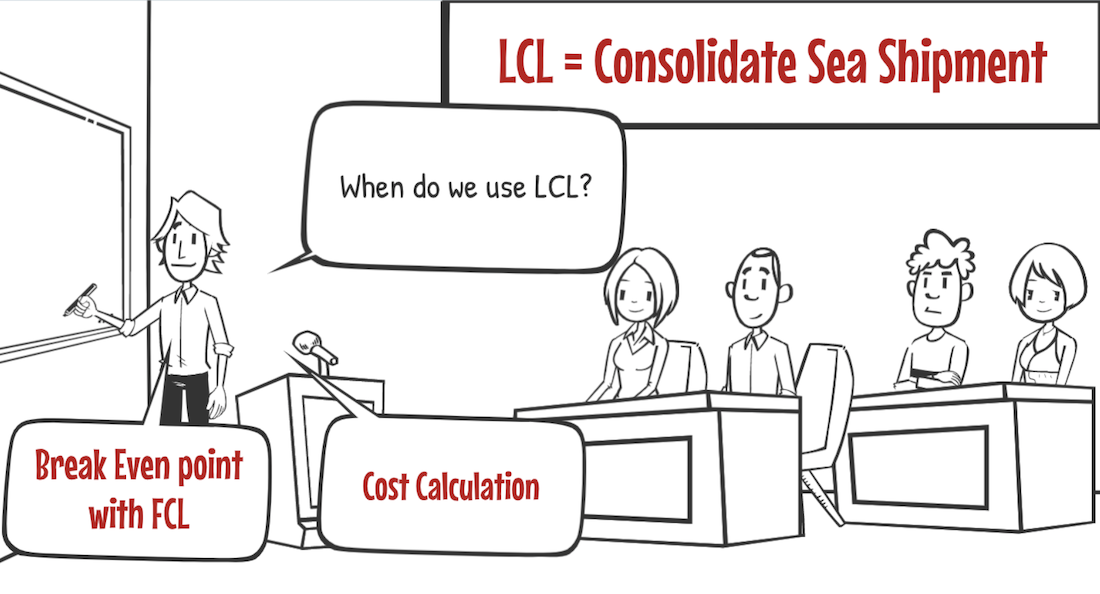LCL is an ocean consolidation service. Unlike FCL, which uses a single container, LCL is a method of transporting cargo by using the space in the container.

So you have to be careful that the cost calculation of LCL is different from FCL. I also explain how to confirm a break-even point and what the maximum cargo volume is to use LCL to be profitable.

## When we should use LCL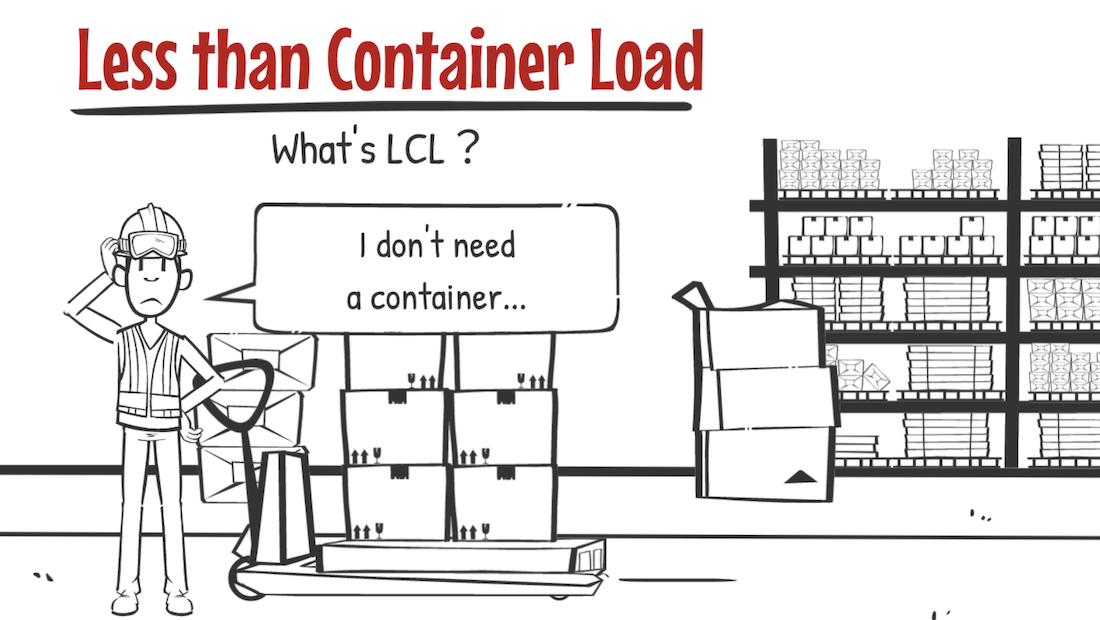First of all, LCL stands for “Less Than Container Loading”. It is used to transport cargo that is less than one container.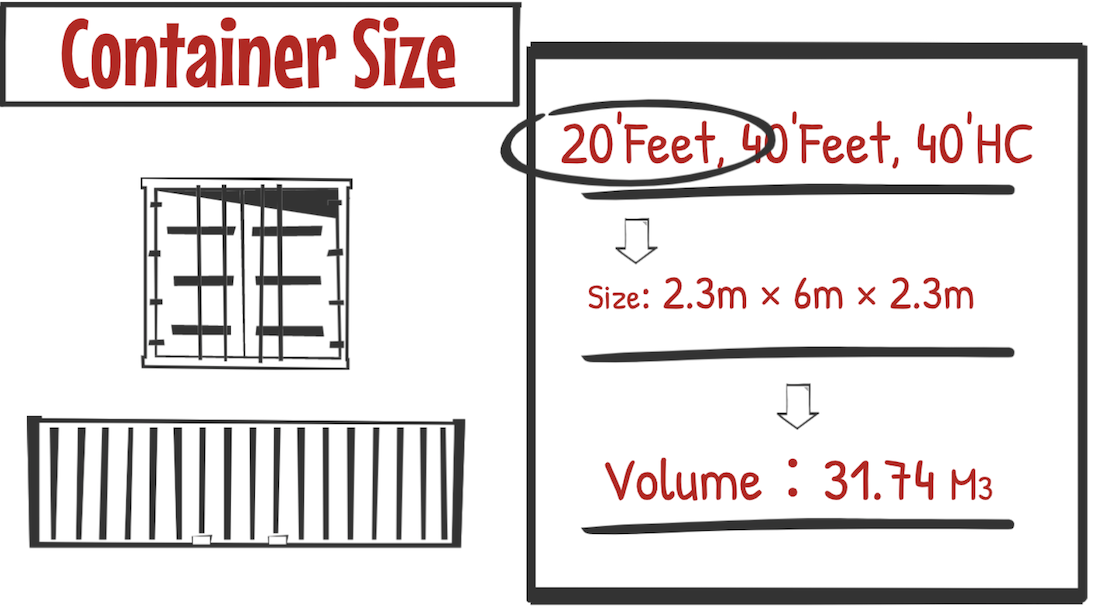LCL is used to transport a cargo that is less than one container. This container here refers to a 20 foot container.

The size of a 20 foot container is about 2.3 meters width, 6 meters length, and 2.3 meters high. The total volume is about 31M3, and with this much volume, a 20 foot container will be filled.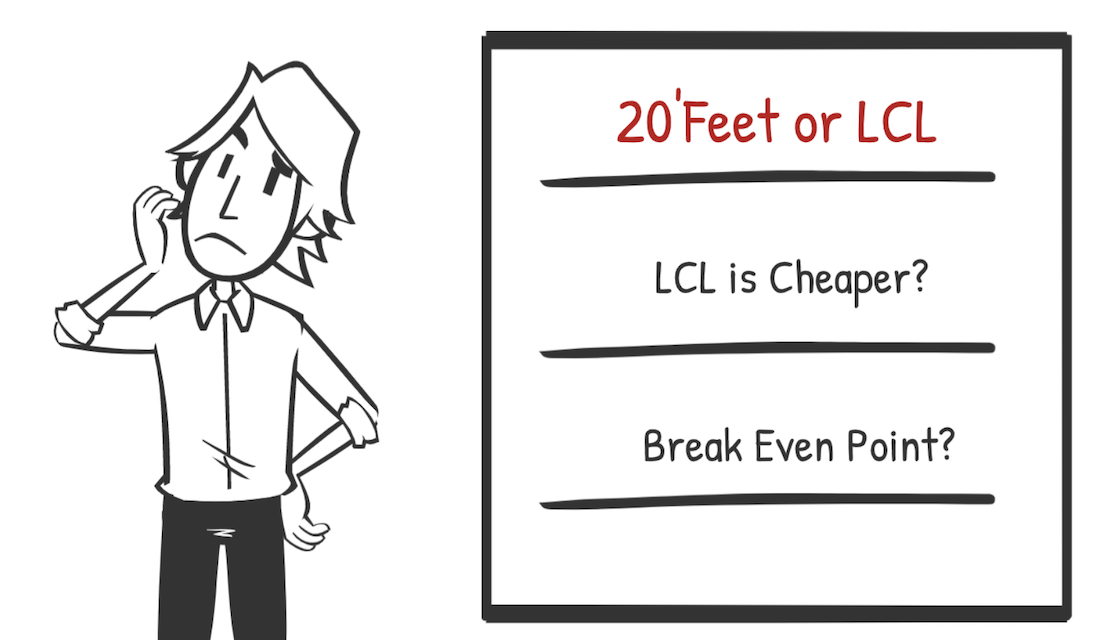Now you know the amount of cargo that fills up a 20 foot container.
But what about when the cargo volume is half of it?

If the cargo volume is 1m3 only, you would use LCL. However, up to how much should you choose LCL?
And how to calculate the break-even point for 20 foot and LCL?

Let’s check it more detail.

## Difference of how to calculate the cost in FCL and LCL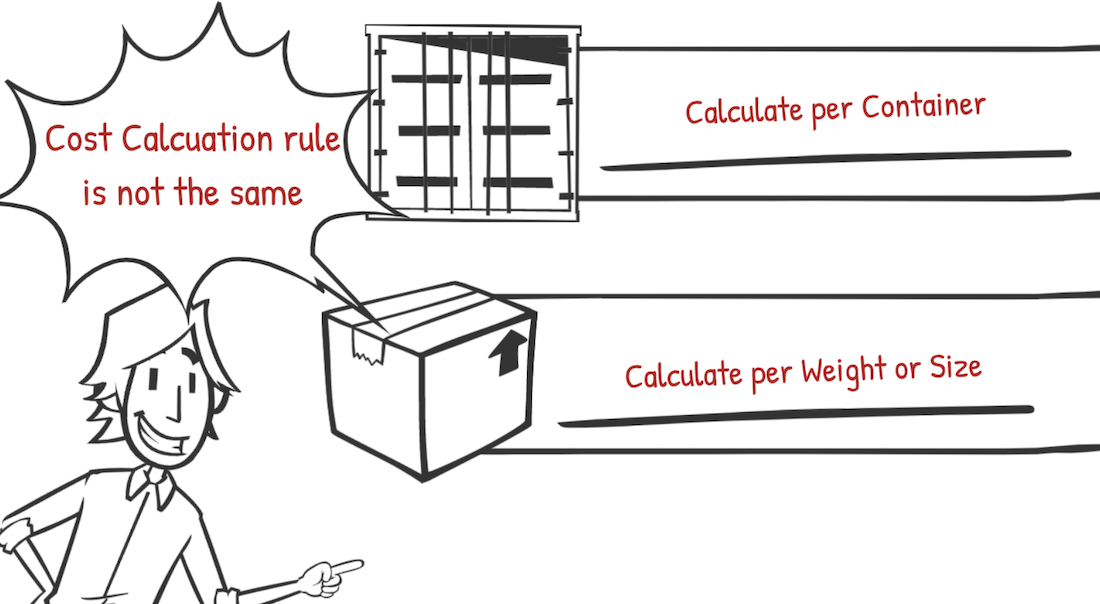Before calculating the break-even point, you have to understand that there are different rules for calculating cost in FCL and LCL.

In contrast to FCL, which calculates the cost per container, LCL converts a charge to size or weight of a cargo, whichever is greater.

Gross Weight and Volume Weight of the cargo are explained in detail in a separate video. I put the link in the description.

### 4 points to compare the cost of FCL and LCL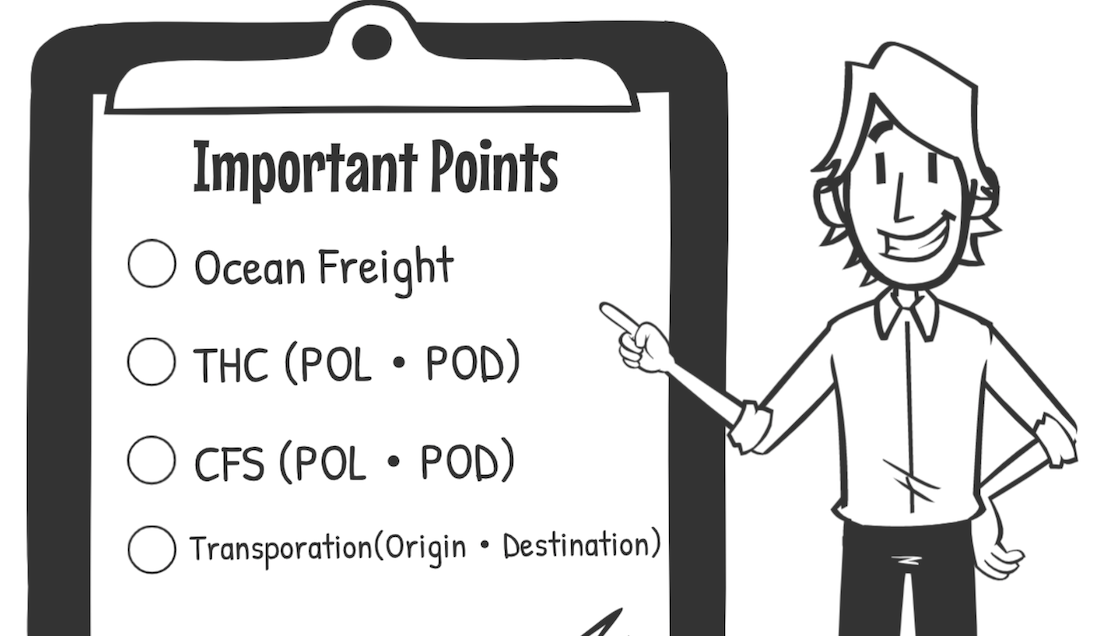When comparing the cost of FCL and LCL, you need to look at these four points. Look at the four main costs: ocean freight, THC, CFS, and truck costs and then, you will find the difference in cost.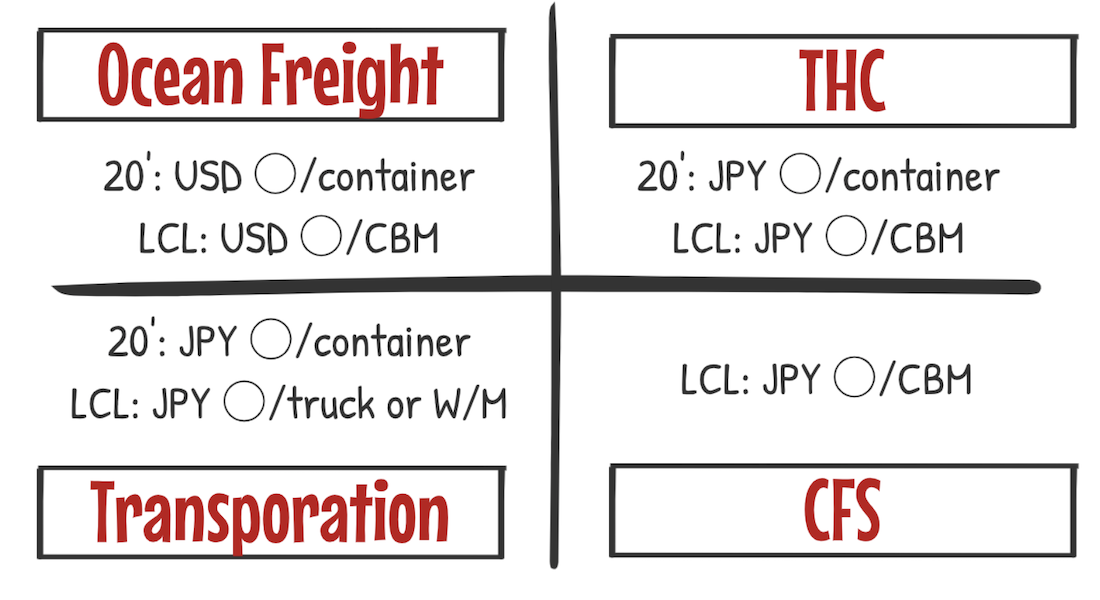Let’s take a closer look. 20 foot FCL has a fixed cost for one container, but LCL will charge for the size or weight of a cargo.

Here, I will explain the unit of shipment by using CBM as the greater size. In this case, CFS is the key point.

CFS refers to cost of a container freight station, where cargo is loaded into a container. It occurs only in LCL. THC is a fee for the using a port, so both FCL and LCL will be charged.

### Example to compare the cost of FCL and LCL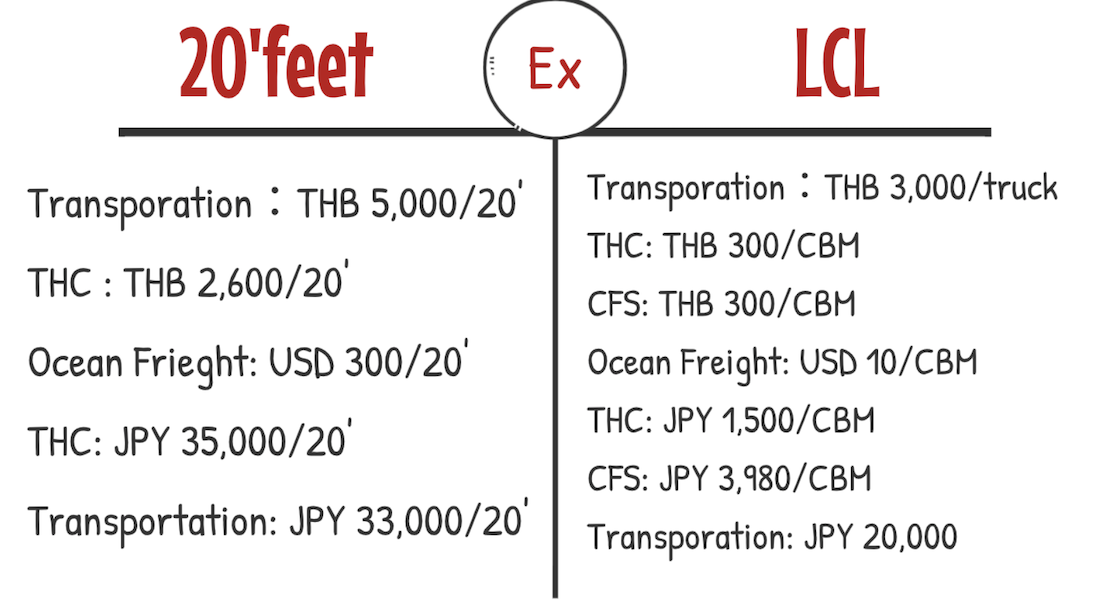Here is an example of a specific number. A lot of numbers come up from here. It may be easier to understand if you stop and watch the video in turn.

Here are some examples of FCL and LCL costs in Bangkok and Tokyo. Ocean freight and trucking fares have different selling prices depending on a forwarder.

But THC and CFS are shipping company’s tariffs, so the cost is roughly like this.

FCL has a fixed cost per 20 foot container, on the other hand, LCL’s ocean freight, THC and CFS vary according to the size of the cargo.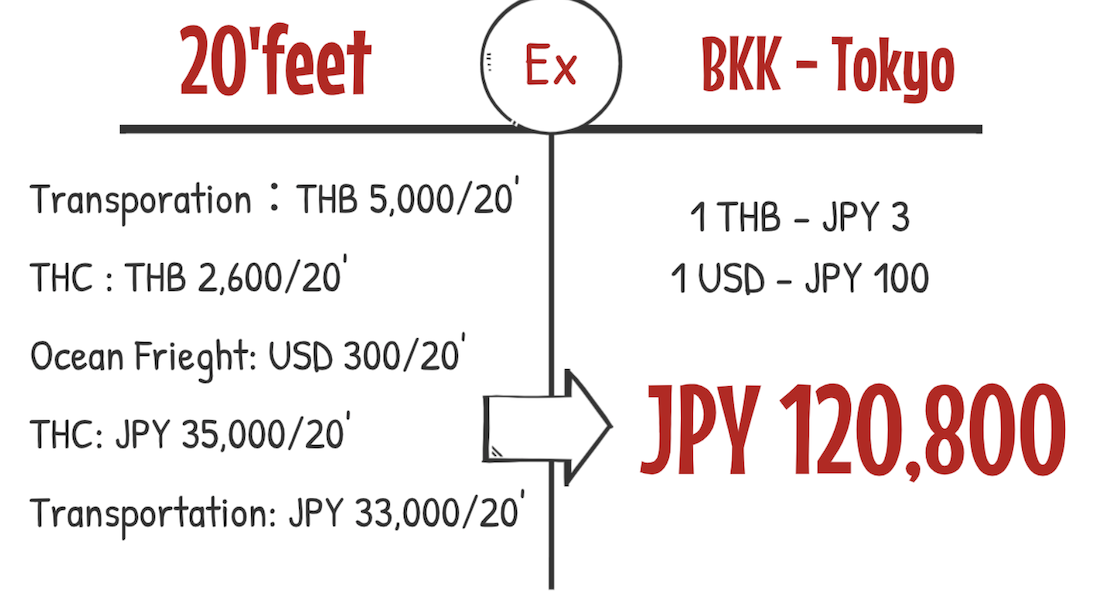To make it easier, let us calculate the total cost. For ease of understanding, assume that 1 baht is 3 yen and 1 dollar is 100 yen. In this case, the cost of one 20 foot container is 120,800 yen.

By the way, this fee does not include custom clearance and documentation fee. Note that this has been simplified to compare the cost of FCL and LCL.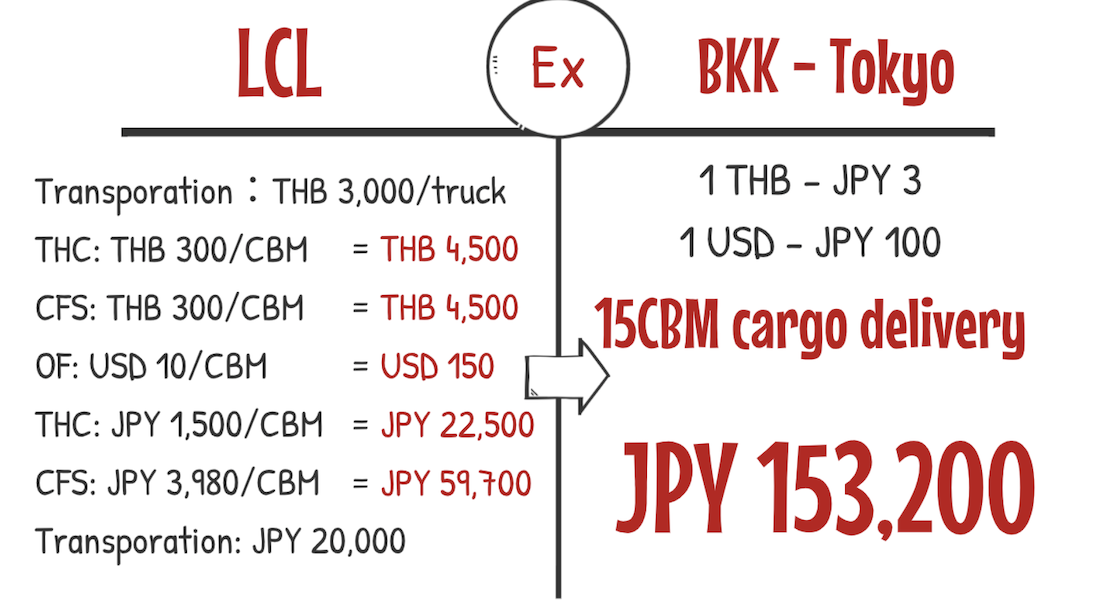On the other hand, assuming that you carry a cargo of 15 CBM by LCL, Since these are costs per CBM, multiply each by 15, then the cost is like this. In total, it is 144,200 yen.Basically, the comparison goes like this. This is in comparison for the case of transporting a 15CBM cargo by LCL.

As the cargo size changes, the cost also changes. And also, it depends on a forwarder’s quotation, FCL may be more expensive.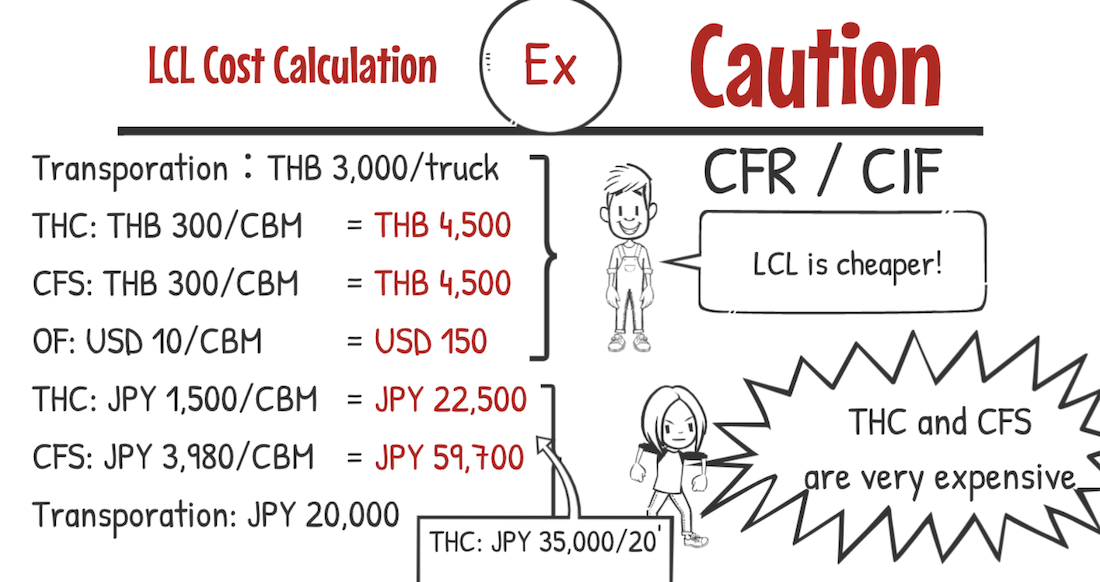### Take care of THC and CFS charge at importing side

Lastly, let’s discuss the most common problems and aware points when sending the cargo by LCL.
On export side, the cost of LCL is generally cheaper than FCL.

Therefore, Shipper who is not used to international transportation, sometimes uses LCL, even if it is a large size cargo. The problem here is the cost of THC and CFS on importing side.

If Incoterms are CFR or CIF, Shipper does not pay for importing side, so if the shipment is large in size but is arranged by LCL, there will be a problem on import side.

If you’re on an export side and arranging a large shipment, Please be careful of calculation, including the cost of importing side by LCL.

## Summary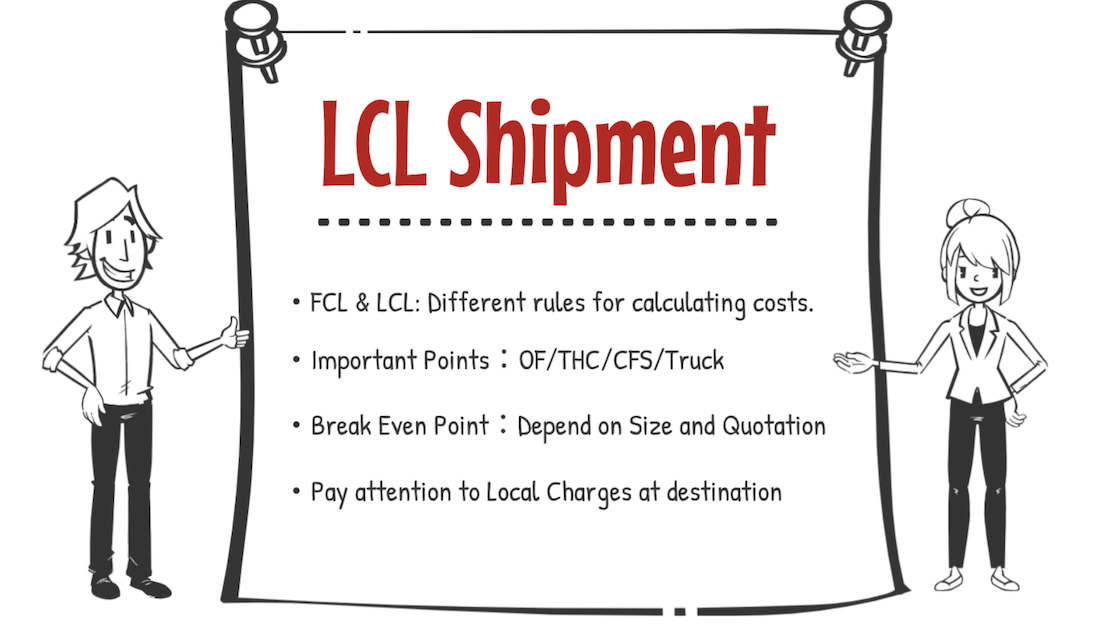LCL is used when a cargo is less than 20 foot container. And please note the following.

FCL and LCL have different rules for calculating costs. In particular, ocean freight, THC, CFS and trucking costs will vary.

The break-even point between FCL and LCL depends on the size of a cargo and the forwarder’s quotation, so, I can’t exactly determine which should be FCL or LCL.

However, when using LCL, it is important to pay attention to THC and CFS of import side. Today’s contents are important to make the best logistics arrangements for the customer.

Please understand it so that you can use it in practice as soon as possible.

## Contact to IINO san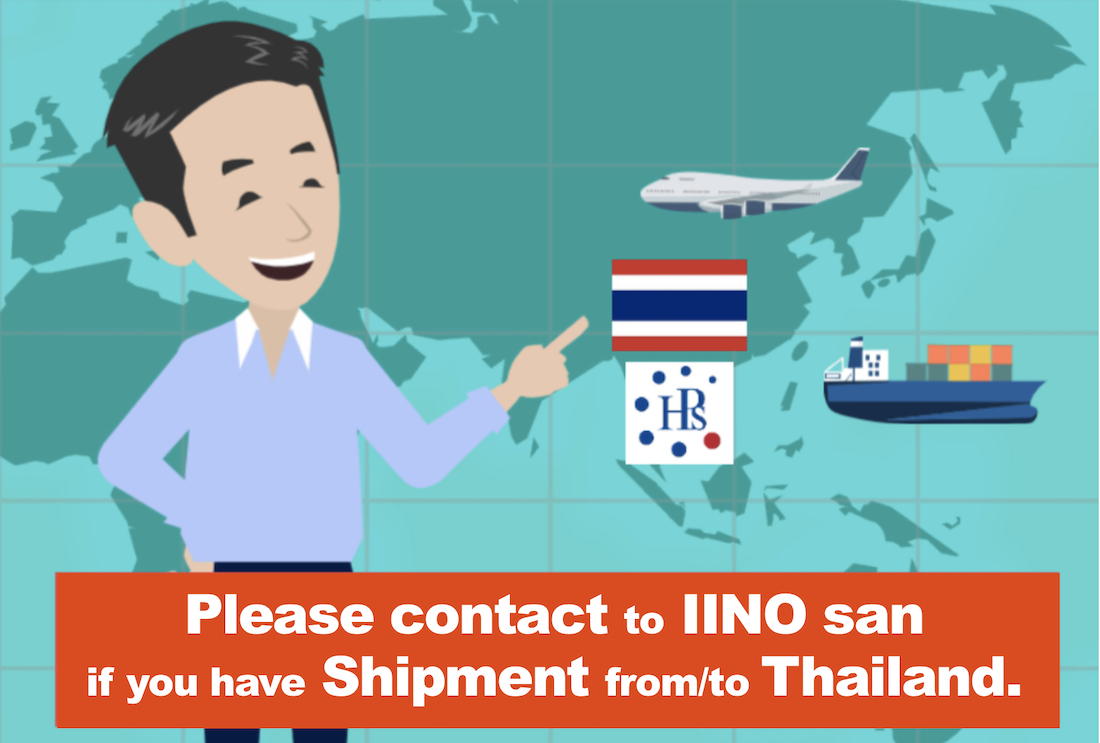★Contact to IINO san★
—————————————–IINO